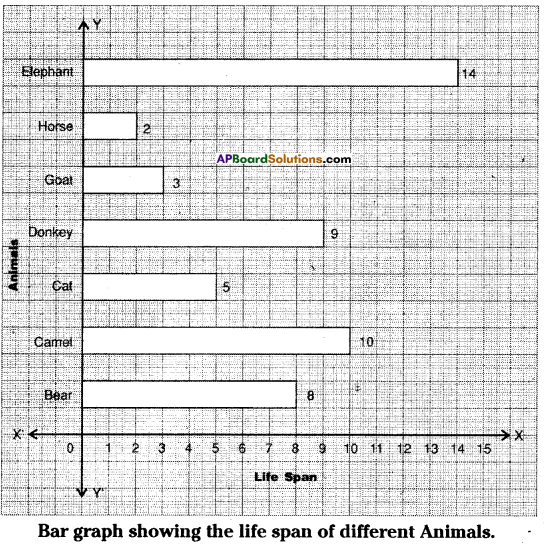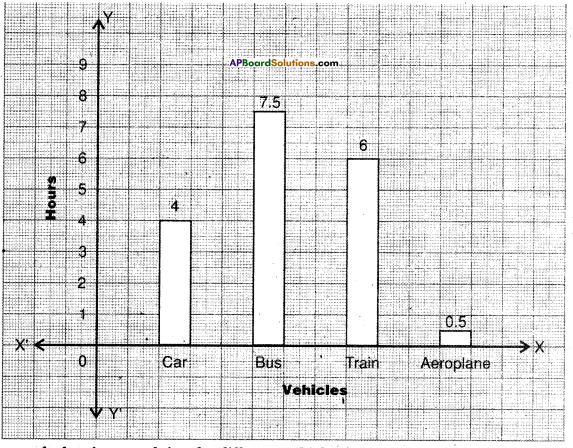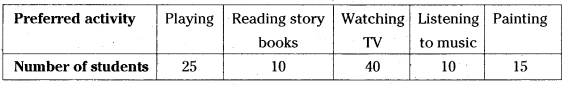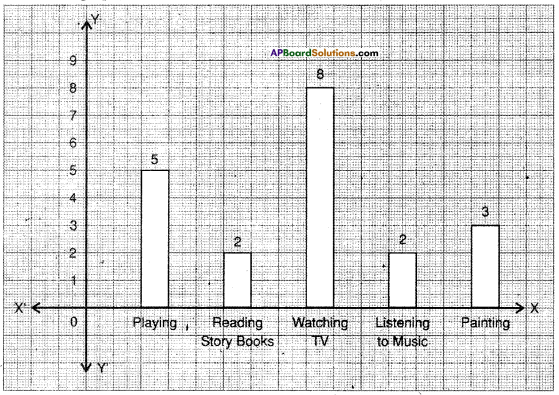# AP Board 6th Class Maths Solutions Chapter 12 Data Handling Ex 12.3

AP State Syllabus AP Board 6th Class Maths Solutions Chapter 12 Data Handling Ex 12.3 Textbook Questions and Answers.

## AP State Syllabus 6th Class Maths Solutions 12th Lesson Data Handling Ex 12.3Question 1.
The life spans of some animals are given below:
Bear – 40 years, Camel – 50 years, Cat – 25 years, Donkey – 45 years, Goat -15 years, Horse -10 years, Elephant – 70 years.
Draw a horizontal bar graph to represent the data.
Solution:Steps of construction:

1. Draw two mutually perpendicular lines on a graph sheet – one horizontal (X-axis) and one vertical (Y – axis).
2. Mark name of the animal on the Y-axis and their life span (Number of years) on the X-axis.
3. Take the GCD or HCF of given numbers as scale on the X-axis i.e., 1cm = 5 years.
4. Calculate lengths or heights of the bars by dividing the life spans with the scale :
Bear = 40 ÷ 5 = 8 cm; Camel = 50 ÷ 5 = 10 cm
Cat = 25 ÷ 5 = 5 cm; Donkey = 45 ÷ 5 = 9 cm
Goat = 15 ÷ 5 = 3 cm; Horse = 10 ÷ 5 = 2 cm
Elephant = 70 ÷ 5 = 14 cm
5. Draw rectangular horizontal bars of same width (1cm) on the Y-axis with their calculated heights.
Hence, required horizontal bar graph was constructed.Question 2.
Travelling time from Hyderabad to Thirupathi by different means of transport are- Car – 8 hours, Bus – 15 hours, Train – 12 hours, Aeroplane – 1 hour. Represent the information using a bar diagram.
Solution:Bar graph showing travel time by different vehicles from Hyderabad to Tirupathi.

Steps of construction :

1. Draw two mutually perpendicular lines on a graph sheet – one horizontal (X-axis) and one vertical (Y-axis).
2. Mark name of the vehicle on the X-axis and their travelling time (Number of hours) on the Y-axis.
3. Take the 2 as scale on the Y-axis i.e., 1 cm = 2 hours.
4. Calculate lengths or heights of the bars by dividing the travelling time with the scale.
Car = 8 ÷ 2 = 4 cm
Bus = 15 ÷ 2 = 7.5 cm
Train = 12 ÷ 2 = 6 cm
Aeroplane = 1 ÷ 2 = 0.5 cm
5. Draw rectangular vertical bars of same width (1cm) on the X-axis, with their calculated heights.
Hence, required vertical bar graph was constructed.Question 3.
A survey of 120 school students was conducted to find which activity they prefer to do in their free time.Draw a bar graph to illustrate the above data.
Solution:Bar graph showing the students can prefer to do Activities in free time.Steps of construction :

1. Draw two mutually perpendicular lines on a graph sheet – one horizontal (X-axis) and one vertical (Y-axis).
2. Mark name of the activity on the X-axis and the number of students on the Y-axis.
3. Take the 5 as scale on the Y-axis i.e., 1cm = 5 students.
4. Calculate length or heights of the bars by dividing the number of students-with the scale.
Playing = 25 ÷ 5 = 5 cm
Reading story books = 10 ÷ 5 = 2 cm
Watching TV = 40 ÷ 5 = 8 cm
Listening to music = 10 ÷ 5 = 2 cm
Painting = 15 ÷ 5 = 3 cm
5. Draw rectangular vertical bars of same width (1cm) on the X-axis with their calculated heights.
Hence, required vertical bar graph was constructed.